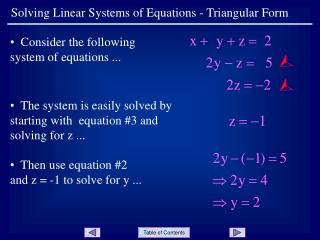DownloadDownload PresentationSolving Linear Systems of Equations - Triangular Form

# Solving Linear Systems of Equations - Triangular Form

Download Presentation## Solving Linear Systems of Equations - Triangular Form

- - - - - - - - - - - - - - - - - - - - - - - - - - - E N D - - - - - - - - - - - - - - - - - - - - - - - - - - -
##### Presentation Transcript

1. Solving Linear Systems of Equations - Triangular Form • Consider the following system of equations ...   • The system is easily solved by starting with equation #3 and solving for z ... • Then use equation #2 and z = -1 to solve for y ...

2. Solving Linear Systems of Equations - Triangular Form • Finally, use equation #1, y = 2 and z = -1 to solve for x ...  • Thus, the solution to the system is (1, 2, -1). • The process just used is called back substitution. Slide 2

3. Solving Linear Systems of Equations - Triangular Form • Now consider the following augmented matrix representing a system of linear equations ... • Note that it is the same system used earlier ... Slide 3

4. Solving Linear Systems of Equations - Triangular Form • If the goal was to solve the system represented by the matrix, we would proceed as before. • Write equation #3 as ... and solve ... • Write equation #2 as ... and solve using z = -1 ... • Write equation #1 as ... and solve using y = 2, z = -2 ... Slide 4

5. Solving Linear Systems of Equations - Triangular Form • The augmented matrix at the right is considered to be in triangular form. The letters a - f represent real numbers. • Along the diagonal, all entries are 1’s. • The bottom left corner forms a triangle of 0’s. • While the 0’s are essential, the author feels that the diagonal of 1’s is not necessary. Often to get the 1’s, fractions are introduced. Slide 5

6. Solving Linear Systems of Equations - Triangular Form • Example: • Use the augmented matrix at the right to solve the system. • Note that the matrix is in triangular form • (not considering the diagonal). • Solve the system using back substitution as before. Slide 6

7. Solving Linear Systems of Equations - Triangular Form • The solution to the system is ... • Note that with an augmented matrix in triangular form, the solution is arrived at very quickly and easily. • So how do we get the triangular form of a matrix? That process is discussed in the presentation titled: • Solving Linear Systems of Equations - Gaussian Elimination Slide 9

8. Solving Linear Systems of Equations - Triangular Form END OF PRESENTATION Click to rerun the slideshow.